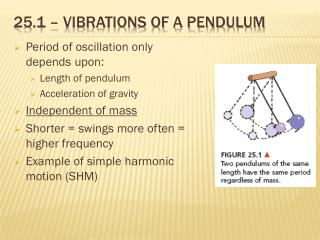DownloadDownload Presentation25.1 – vibrations of a pendulum

# 25.1 – vibrations of a pendulum

Download Presentation## 25.1 – vibrations of a pendulum

- - - - - - - - - - - - - - - - - - - - - - - - - - - E N D - - - - - - - - - - - - - - - - - - - - - - - - - - -
##### Presentation Transcript

1. 25.1 – vibrations of a pendulum • Period of oscillation only depends upon: • Length of pendulum • Acceleration of gravity • Independent of mass • Shorter = swings more often = higher frequency • Example of simple harmonic motion (SHM)

2. Masses on springs (honors) • Masses on spring also exhibit SHM • Restoring force α distance from equilibrium • Bigger k = stiffer spring • Oscillating spring systems only depend upon: • Mass at end of spring • Spring constant

3. 25.2 – wave description • Vibrations are what produces waves • Looks like a sine wave • # of cycles (vibrations) per second = frequency (f) • Unit: s-1 = hertz (Hz) • Period (T) = time for 1 cycle

4. 25.3 – wave motion • Waves transfer energy not matter • Only temporary motion of matter • No matter is transmitted between 2 points • The matter “bangs” into matter next to it, giving it energy

5. 25.4 – wave speed • Depends upon medium • Can be calculated as the distance a crests moves in a certain time • Fundamental relationship between: speed, wavelength & frequency • For the same type of wave – speed is the same • λ & f are inverses of one another

6. 25.5 & 25.6 – transverse & longitudinal waves • Wave pulse is perpendicular (across) from the direction of travel • EM waves need no medium to travel • Oscillation is back and forth in the direction of wave travel • Sound waves

7. Electromagnetic waves • A self propagation of E & B fields • As one changes, so must the other • Moves at the speed of light, c = 3.00 x 108 m/s • Caused because of accelerating electric charges

8. Vibrations determine the frequency of EM waves • Visible light is just a sliver of EM spectrum • Includes: radio waves, microwaves, infrared, visible, ultraviolet, x-rays, gamma rays • In order of increasing energy

9. 25.7 - interference • When waves meet and overlap • Constructive interference • Waves meet & amplitude gets larger • Destructive interference • Amplitude gets smaller • When wave crests exactly line up – in phase • Not common • Out of phase when crest & trough overlap • Creates dark bands

10. 25.8 – standing waves • Waves generated have locations that appear to not move • Parts of wave that appear stationary – nodes • Complete destructive interference • Next to these are locations of maximum amplitude – antinodes – constructive int. • As waves meet, they interfere and then pass through one another • Higher frequency generate more standing waves

11. 25.9 – doppler effect • The apparent change in frequency due to motion of source or observer • Waves move in all directions at same speed • Source moving “bunches up” waves in direction of motion & “spreads out” behind

12. Frequency is higher in direction of motion – a higher pitch • Occurs for all waves – sound & light • Blue shift – object towards us • Red shift - away

13. 25.10 – bow waves • Bow waves occur when wave source moves faster than the waves produced

14. 25.11 – shock waves • Shock waves are produced when object is faster than speed of sound • Caused because of constructive interference • Creates a conical shell of compressed air • This is the sonic boom • Always carried with plane (object) going ≥ vsound